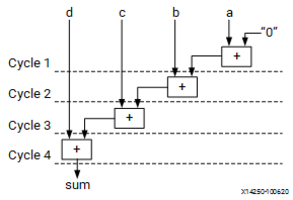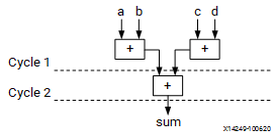# Optimizing Logic Expressions - 2022.1 English

## Vitis High-Level Synthesis User Guide (UG1399)

Document ID
UG1399
Release Date
2022-06-07
Version
2022.1 English

During synthesis several optimizations, such as strength reduction and bit-width minimization are performed. Included in the list of automatic optimizations is expression balancing.

Expression balancing rearranges operators to construct a balanced tree and reduce latency.

• For integer operations expression balancing is on by default but may be disabled using the EXPRESSION_BALANCE pragma or directive.
• For floating-point operations, expression balancing is off by default but may be enabled using using the ```config_compile -unsafe_math_optimizations``` command, as discussed below.

Given the highly sequential code using assignment operators such as `+=` and `*=` in the following example (or resulting from loop unrolling):

``````data_t foo_top (data_t a, data_t b, data_t c, data_t d)
{
data_t sum;

sum = 0;
sum += a;
sum += b;
sum += c;
sum += d;
return sum;

}``````

Without expression balancing, and assuming each addition requires one clock cycle, the complete computation for `sum` requires four clock cycles shown in the following figure.

Figure 1. Adder TreeHowever additions `a+b` and `c+d` can be executed in parallel allowing the latency to be reduced. After balancing the computation completes in two clock cycles as shown in the following figure. Expression balancing prohibits sharing and results in increased area.

Figure 2. Adder Tree After BalancingFor integers, you can disable expression balancing using the EXPRESSION_BALANCE optimization directive with the `off` option. By default, Vitis HLS does not perform the EXPRESSION_BALANCE optimization for operations of type `float` or `double`. When synthesizing `float` and `double` types, Vitis HLS maintains the order of operations performed in the C/C++ code to ensure that the results are the same as the C/C++ simulation. For example, in the following code example, all variables are of type `float` or `double`. The values of `O1` and `O2` are not the same even though they appear to perform the same basic calculation.

``````A=B*C; A=B*F;
D=E*F; D=E*C;
O1=A*D O2=A*D;``````

This behavior is a function of the saturation and rounding in the C/C++ standard when performing operation with types `float` or `double`. Therefore, Vitis HLS always maintains the exact order of operations when variables of type `float` or `double` are present and does not perform expression balancing by default.

You can enable expression balancing for specific operations, or you can configure the tool to enable expression balancing with `float` and `double` types using the ```config_compile -unsafe_math_optimizations``` command as follows:

1. In the Vitis HLS IDE, select Solution > Solution Settings.
2. In the Solution Settings dialog box, click the General category, select config_compile, and enable unsafe_math_optimizations.

With this setting enabled, Vitis HLS might change the order of operations to produce a more optimal design. However, the results of C/RTL co-simulation might differ from the C/C++ simulation.

The `unsafe_math_optimizations` feature also enables the `no_signed_zeros` optimization. The `no_signed_zeros` optimization ensures that the following expressions used with `float` and `double` types are identical:

``````x - 0.0 = x;
x + 0.0 = x;
0.0 - x = -x;
x - x = 0.0;
x*0.0 = 0.0;``````

Without the `no_signed_zeros` optimization the expressions above would not be equivalent due to rounding. The optimization may be optionally used without expression balancing by selecting only this option in the `config_compile` command.

Tip: When the `unsafe_math_optimizations` and `no_signed_zero` optimizations are used, the RTL implementation will have different results than the C/C++ simulation. The test bench should be capable of ignoring minor differences in the result: check for a range, do not perform an exact comparison.